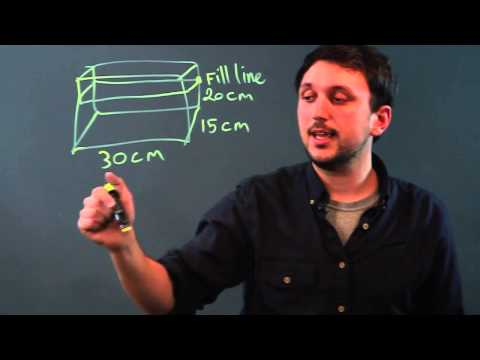## Video: Measuring And Calculating Aquarium VolumesVideo: What Is the Volume of Water in a Fish Tank in Liters? 2023, September

## What does 20% of aquarium water mean?

Let's look at some examples. If you have a 45 liter aquarium, 20% of 45 liters = 0.2x45 = 9 liters. Take a regular measuring cup, for example 0.5L, and fill in 18 cups of water, which will be 20%. Now mark the water level in your tank with a marker or some other method. In the future, you can navigate by this label.

Another example. You don't know how many liters you have in your tank. Take a ruler or measuring tape, measure the height of the vertical side of the aquarium (from the bottom to the water level) and multiply it by 20%. For example, most small aquariums are approximately 30 cm high and the water level reaches 24 cm. In this case, 20% of 24 cm = 0.2x24 = 4.8 cm. Measure 4.8 cm from the water level and mark this place with a marker on the surface of the aquarium.

Remember that replacing 20% of the water with tap water is usually safe even without special conditioners, and replacing more water can harm the inhabitants of the aquarium, even when using special preparations. In this case, it is best to replace 20% of the water twice a week.

## How many liters are in my aquarium?

Many medicines and preparations contain recommendations to add a certain amount of funds for a certain amount of water. Therefore, it is very important to know the exact volume of the aquarium. If you do not know it, use our advice and calculate it yourself.

Measure the aquarium from left to right. For example, let its length be 45 cm.

Measure your aquarium from bottom to top (high). For example, let's take a height of 30 cm.

Now measure the width of the aquarium (front to back). For example, the width is 30 cm.

Then multiply these three dimensions. In our example, we get 45x30x30 = 40500 cm 3. Now this number must be multiplied by 0.001 to get liters. In our example, we get 40500x0.001 = 40.5 liters.

## Small amendment

Almost every aquarium contains large rocks, a layer of gravel, and other objects that reduce the amount of water in the aquarium. Of course, you can collect all these objects, place them in a basin of a known volume and try to calculate the volume of all these objects by manipulating the water. All these calculations are quite complicated, but at the same time very interesting. So if you have plenty of time to spare, you can try and measure the exact volume of water in your tank. Nevertheless, in most cases it is sufficient to use the formula that was given above.